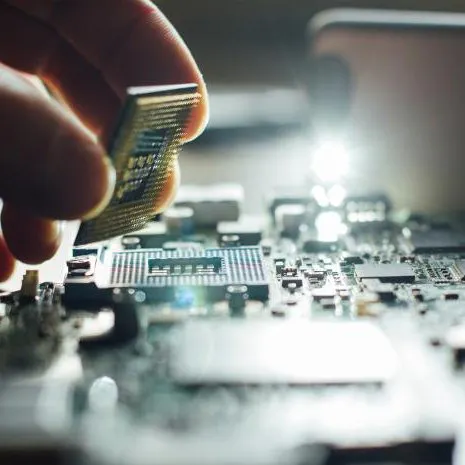Published

# ESP8266 Clock Using MAX7219 LED Matrix Display

A simple connected clock and weather report using ESP8266, MAX7219 LED matrix display, and DHT11 temperature and humidity sensor.

IntermediateFull instructions provided1 hour11,953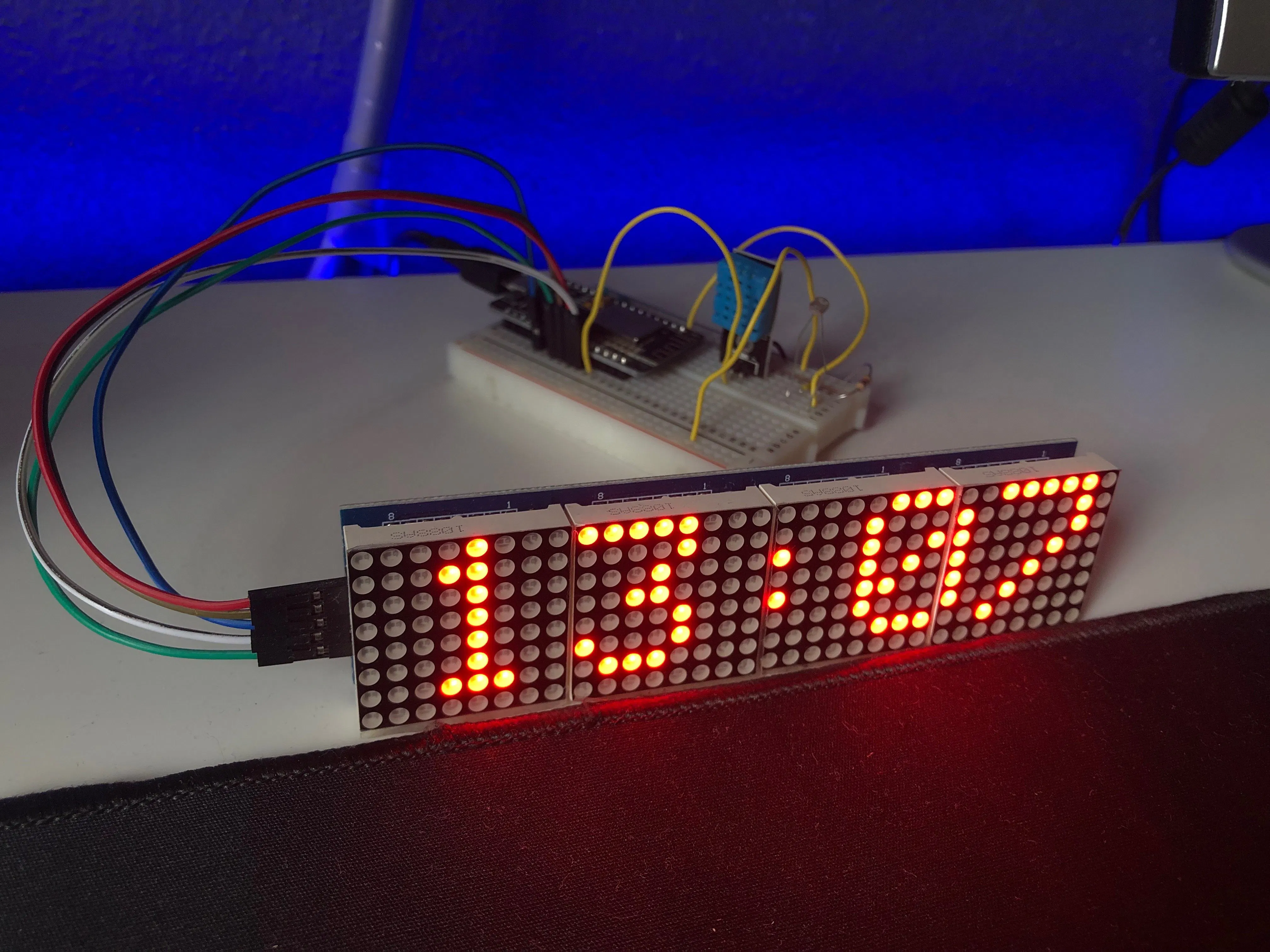## Things used in this project

### Hardware components

 ESP8266 NodeMCU
×1
 MAX7219 LED Matrix Display
×1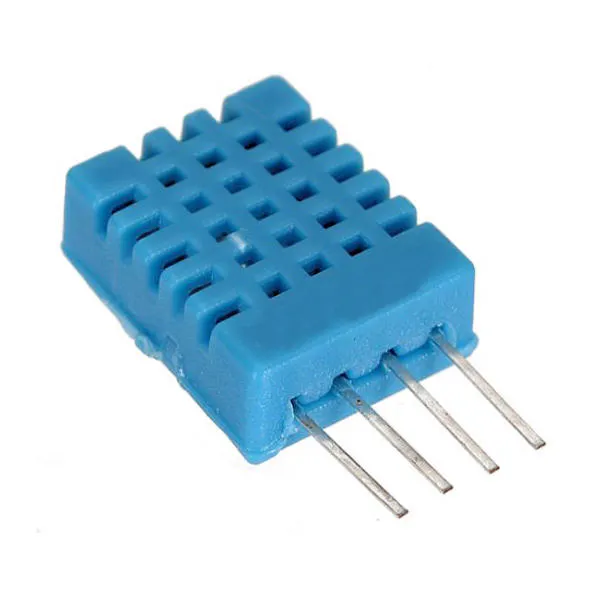DHT11 Temperature & Humidity Sensor (4 pins)
×1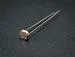Photo resistor
×1×1Jumper wires (generic)
×1

### Software apps and online servicesArduino IDE

## Schematics

### Clock_Schematics.png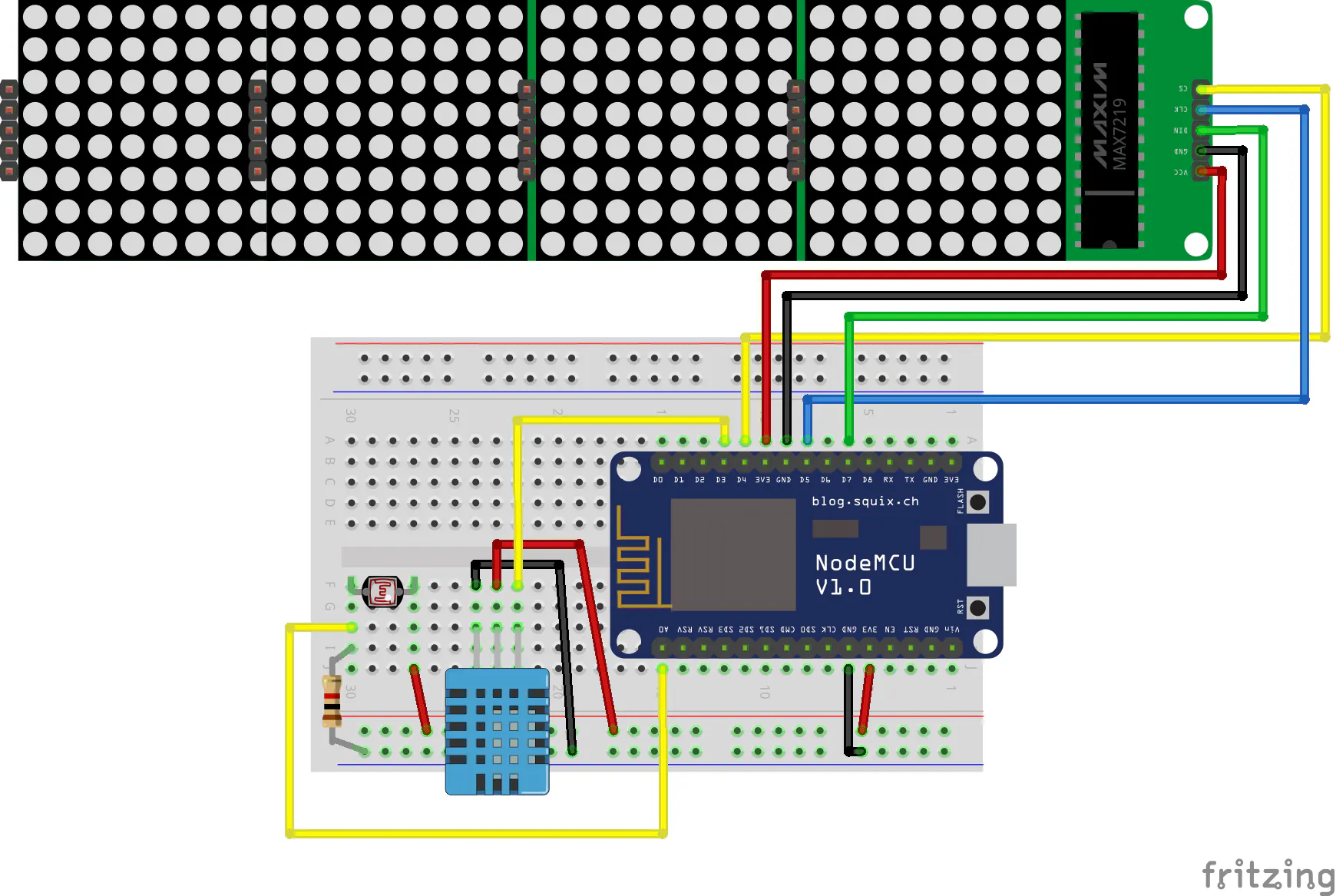## Code

### Clock_Code.ino

C/C++
```#include <ESP8266WiFi.h>
#include <SPI.h>
#include <Max72xxPanel.h>
#include <time.h>
#include "DHT.h"

int pinCS = D4;
int numberOfHorizontalDisplays = 4;
int numberOfVerticalDisplays   = 1;
char time_value;

// LED Matrix Pin -> ESP8266 Pin
// Vcc            -> 3v  (3V on NodeMCU 3V3 on WEMOS)
// Gnd            -> Gnd (G on NodeMCU)
// DIN            -> D7  (Same Pin for WEMOS)
// CS             -> D4  (Same Pin for WEMOS)
// CLK            -> D5  (Same Pin for WEMOS)

Max72xxPanel matrix = Max72xxPanel(pinCS, numberOfHorizontalDisplays, numberOfVerticalDisplays);

int wait = 70; // In milliseconds

int spacer = 1;
int width  = 5 + spacer; // The font width is 5 pixels

int m;

#define DHTPIN 0          // D3

#define DHTTYPE DHT11     // DHT 11

DHT dht(DHTPIN, DHTTYPE);

String t, h;

void setup() {
Serial.begin(9600);
dht.begin();

//CHANGE THE POOL WITH YOUR CITY. SEARCH AT https://www.ntppool.org/zone/@

configTime(0 * 3600, 0, "it.pool.ntp.org", "time.nist.gov");

setenv("TZ", "GMT-1BST",1);

matrix.setIntensity(0); // Use a value between 0 and 15 for brightness
matrix.setRotation(0, 1);    // The first display is position upside down
matrix.setRotation(1, 1);    // The first display is position upside down
matrix.setRotation(2, 1);    // The first display is position upside down
matrix.setRotation(3, 1);    // The first display is position upside down
matrix.fillScreen(LOW);
matrix.write();

while ( WiFi.status() != WL_CONNECTED ) {
matrix.drawChar(2,0, 'W', HIGH,LOW,1); // H
matrix.drawChar(8,0, 'I', HIGH,LOW,1); // HH
matrix.drawChar(14,0,'-', HIGH,LOW,1); // HH:
matrix.drawChar(20,0,'F', HIGH,LOW,1); // HH:M
matrix.drawChar(26,0,'I', HIGH,LOW,1); // HH:MM
matrix.write(); // Send bitmap to display
delay(250);
matrix.fillScreen(LOW);
matrix.write();
delay(250);
}
}

void loop() {
matrix.setIntensity(m);
matrix.fillScreen(LOW);
time_t now = time(nullptr);
String time = String(ctime(&now));
time.trim();
//Serial.println(time);
time.substring(11,19).toCharArray(time_value, 10);
matrix.drawChar(2,0, time_value, HIGH,LOW,1); // H
matrix.drawChar(8,0, time_value, HIGH,LOW,1); // HH
matrix.drawChar(14,0,time_value, HIGH,LOW,1); // HH:
matrix.drawChar(20,0,time_value, HIGH,LOW,1); // HH:M
matrix.drawChar(26,0,time_value, HIGH,LOW,1); // HH:MM
matrix.write(); // Send bitmap to display

delay(30000);

matrix.fillScreen(LOW);
display_message(t+"C "+h+"%");
}

void display_message(String message){
for ( int i = 0 ; i < width * message.length() + matrix.width() - spacer; i++ ) {
//matrix.fillScreen(LOW);
int letter = i / width;
int x = (matrix.width() - 1) - i % width;
int y = (matrix.height() - 8) / 2; // center the text vertically
while ( x + width - spacer >= 0 && letter >= 0 ) {
if ( letter < message.length() ) {
matrix.drawChar(x, y, message[letter], HIGH, LOW, 1); // HIGH LOW means foreground ON, background off, reverse to invert the image
}
letter--;
x -= width;
}
matrix.write(); // Send bitmap to display
delay(wait/2);
}
}
```

## Credits

### FilippoOnesti

1 project • 0 followers
Computer Engineering Student in Ferrara, Italy. Arduino | Raspberry | IoT Python, Java, HTML, CSS